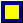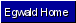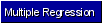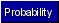Egwald Statistics: Probability and Stochastic Processes by Elmer G. Wiens Egwald's popular web pages are provided without cost to users. Please show your support by joining Egwald Web Services as a Facebook Fan:Follow Elmer Wiens on Twitter:Normal Distribution Samples A. Sample Mean Suppose a random variable X has the normal distribution N(µ, ø^2) and {X1, ...Xn} is a sample of n observations of X. Then, the random variable Y defined by Y = (X1 + ... + Xn)/n is called the sample mean. The r.v. Y has normal distribution N(µ, ø^2/n). Density of the Sample Mean With µ = 0 ,ø^2 = 4, and n = 10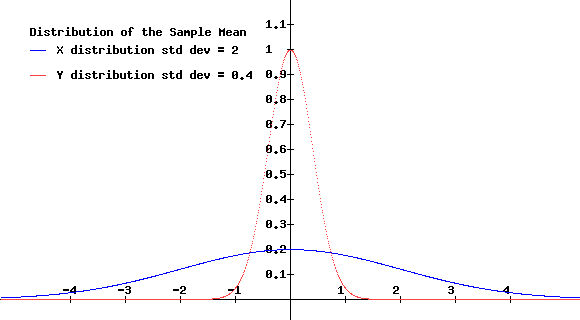B. Sample Variance The random variable S^2 defined by S^2 = [(X1 - Y)^2 + ... + (Xn - Y)^2] / (n - 1) is called the sample variance. S is called the standard error of the mean. The r.v. (n - 1) * S^2 / ø^2 has the chi-square distribution with n - 1 degrees of freedom. Suppose that from a sample of ten observations of the population r.v. X, we find that S^2 = 7.6. Suppose, further, we want to test if the population normal r.v. X has variance ø^2 = 4. Then (n - 1) * S^2 / ø^2 = 9 * 7.6 / 4 = 17.1 From the graph below, 17.1 > 16.919, so we can reject, with considerable confidence, the hypotheses that the population r.v. X has a variance of 4.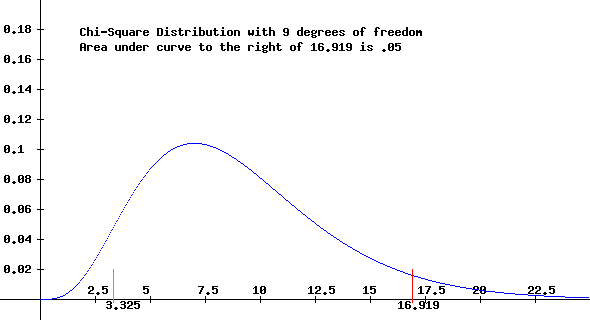Copyright © Elmer G. Wiens:   Egwald Web ServicesAll Rights Reserved.    Inquiries